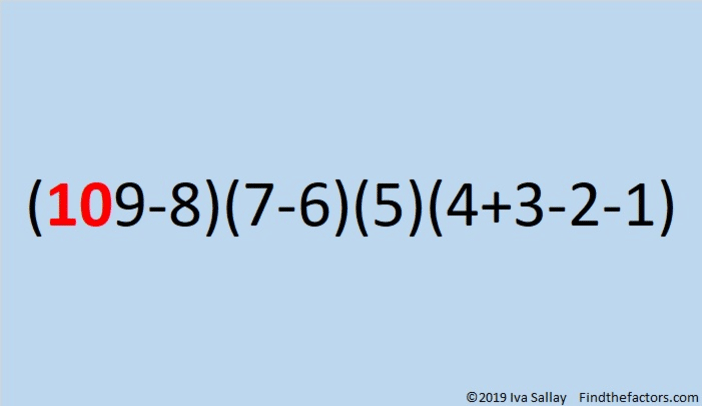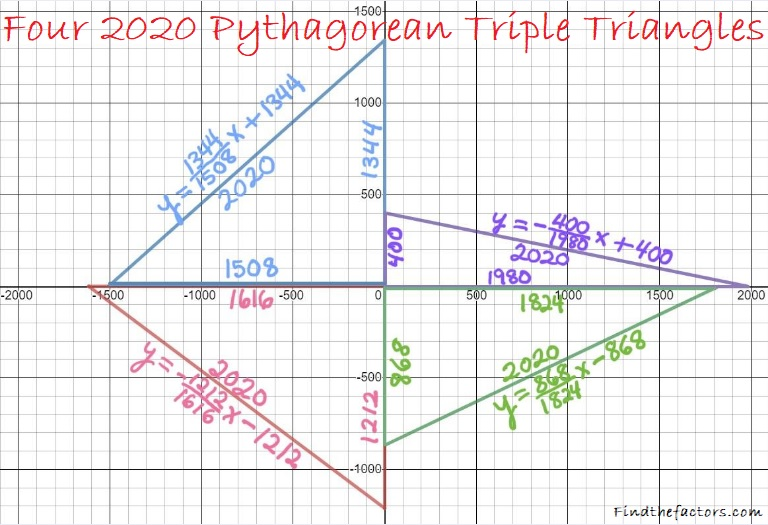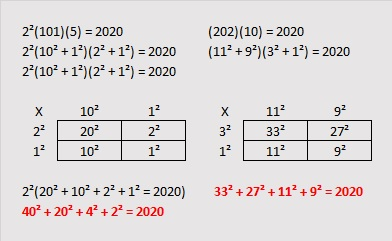# Countdown to 2020

Contents

#### Countdown to 2020:

It seems that every New Year’s Eve, mathematicians come up with equations with the new year in it. Some of those equations will be a countdown. Here is the equation that I found and made into a gif:make science GIFs like this at MakeaGif

#### Factors of 2020:

What will the factors be in the year 2020? I’m here ready with my predictions and the reasons that you can rely on them:
• 2020 is a composite number.
• Prime factorization: 2020 = 2 × 2 × 5 × 101, which can be written 2020 = 2² × 5 × 101
• 2020 has at least one exponent greater than 1 in its prime factorization so √2020 can be simplified. Taking the factor pair from the factor pair table below with the largest square number factor, we get √2020 = (√4)(√505) = 2√505
• The exponents in the prime factorization are 2, 1, and 1. Adding one to each exponent and multiplying we get (2 + 1)(1 + 1)(1 + 1) = 3 × 2 × 2 = 12. Therefore 2020 has exactly 12 factors.
• The factors of 2020 are outlined with their factor pair partners in the graphic below.

#### A 2020 Factor Tree and a 2020 Factor Cake:

A 2020 factor tree may interest you:

Or a 2020 Factor Cake:

#### Other Facts about the number 2020:

2020 is palindrome 4C4 in BASE 21 (C is 12 in base 10):
4(21²) + 12(21) + 4(1) = 4(441) + 12(21) + 4 = 1764 + 252 + 4 = 2020

42² + 16² = 2020
38² + 24² = 2020

2020 is the hypotenuse of FOUR Pythagorean triples:
400-1980-2020 which is 20 times (20-99-101)
868-1824-2020 calculated from 38² – 24², 2(38)(24), 38² + 24²
1212-1616-2020 which is (3-4-5) times 404
1344-1508-2020 calculated from 2(42)(16), 42² – 16², 42² + 16²2020 is the difference of two squares two different ways:
506² – 504² = 2020
106² – 96² = 2020
Can you calculate when 2020 is a leg in a Pythagorean triple from those equations? (The Pythagorean triples can be calculated from a² – b², 2(a)(b),  a² + b²)

Then can you calculate other times 2020 is a leg in a Pythagorean triple from these facts? (The Pythagorean triples can be calculated from 2(a)(b), a² – b²,  a² + b²)
2(1010)(1) = 2020
2(505)(2) = 2020
2(202)(5) = 2020
2(101)(10) = 2020
Did any of those equations produce the same Pythagorean triples that the difference of two squares produced?

2020 is the sum of four squares in MANY different ways. Here is how I found two of those ways: